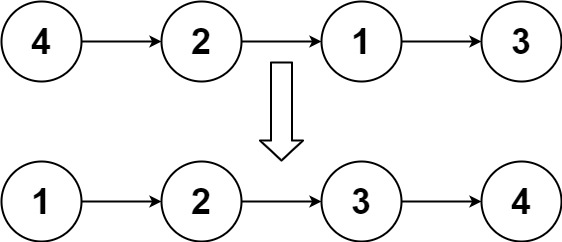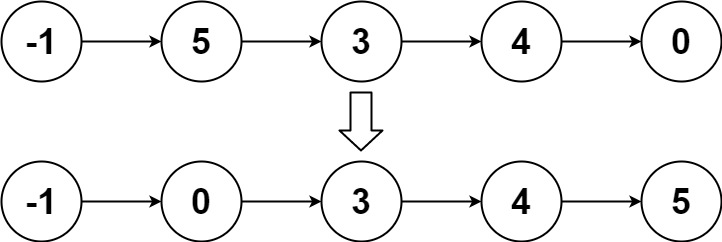# LeetCode: 148. Sort List

## 题目¶

Given the head of a linked list, return the list after sorting it in ascending order.

Follow up: Can you sort the linked list in O(n logn) time and O(1) memory (i.e. constant space)?

Example 1:```Input: head = [4,2,1,3]
Output: [1,2,3,4]
```

Example 2:```Input: head = [-1,5,3,4,0]
Output: [-1,0,3,4,5]
```

Example 3:

```Input: head = []
Output: []
```

Constraints:

• The number of nodes in the list is in the range [0, 5 * 10^4].
• -10^5 <= Node.val <= 10^5

## 解法¶

### 使用归并排序的方法对链表进行排序¶

• 递归切分链表为两部分，分别对两部分进行排序，然后再合并排序过后的各个部分。
• 二分链表需要找到链表中间元素，可以通过快慢指针的方法寻找：慢指针每次移动一步，快指针每次移动两步，当快指针到达链表尾部时，慢指针刚好在链表中间位置。
• 合并排序后的部分可以参考前面的 LeetCode: 21. Merge Two Sorted Lists

```# Definition for singly-linked list.
# class ListNode(object):
#     def __init__(self, val=0, next=None):
#         self.val = val
#         self.next = next
class Solution(object):

right = self.sortList(mid)

pre = None
while fast is not None and fast.next is not None:
pre = slow
slow = slow.next
fast = fast.next.next

# 通过 pre.next=None 将链表从中间切断为两个链表
if pre is not None:
pre.next = None
return slow

dummy = ListNode()
tail = dummy
while l1 is not None and l2 is not None:
if l1.val < l2.val:
tail.next = l1
l1 = l1.next
else:
tail.next = l2
l2 = l2.next
tail = tail.next

if l1 is not None:
tail.next = l1
if l2 is not None:
tail.next = l2

return dummy.next
```